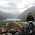## Monday, September 9, 2013

### Plotting facing trees using phytools

A recent R-SIG-phylo user asked how to plot "mirror" or facing trees. This is pretty straightforward, in general. For instance, we can use cophylo.plot in the ape package to draw two facing trees in the same plot. However, there are other options as well - especially using par(mfrow) and layout. Here's a quick demo of how we can use these functions to plot facing phylogenies in their simplest forms:

> ## load phytools
> require(phytools)
> packageVersion("phytools")
 ‘0.3.47’
> ## simulate a tree & make realistic looking tip labels
> tree<-pbtree(n=26)
> tree\$tip.label<-sapply(LETTERS[26:1],function(x) paste(x,".",paste(sample(letters,round(runif(n=1,min=4,v max=10))),collapse=""),sep=""))
> ## here is that tree on its own
> plotTree(tree)
> ## now let's try facing trees in which we repeat the
> ## tip labels
> par(mfrow=c(1,2))
> plotTree(tree)
> plotTree(tree,direction="leftwards")

If our tip labels are the same & in the same order in the two trees, we can plot the labels only once using the function layout to split our plotting area into three parts: two for the trees with no labels, and a third (the middle segment) for our centered tip labels.

> ## now let's do it writing the tip labels only once
> ## we'll have to adjust widths based on the size of our > ## labels
> layout(matrix(1:3,1,3),widths=c(0.42,0.16,0.42))
> plotTree(tree,ftype="off")
> plot.new()
> plot.window(xlim=c(-0.1,0.1),ylim=c(1, length(tree\$tip.label)))
> par(cex=1)
> text(rep(0,length(tree\$tip.label)), 1:length(tree\$tip.label),tree\$tip.label)
> plotTree(tree,ftype="off",direction="leftwards")

The neatest thing, of course, is if we use the same approach to show the result of some of phytools custom visualizations. For instance, let's show a "contMap" plot of two different correlated characters in each of the subplots, as follows. (Note that this uses a new version of contMap from the latest non-CRAN phytools release, phytools 0.3-47.)

> ## simulate correlated trait data
> V<-matrix(c(1,0.8,0.8,1),2,2)
> X<-sim.corrs(tree,V)
> layout(matrix(1:3,1,3),widths=c(0.44,0.12,0.44))
> par(cex=1)
> contMap(tree,X[,1],ftype="off",sig=1,legend=1)
> ylim<-c(1-0.12*(length(tree\$tip.label)-1),length(tree\$tip.label))
> plot.new(); plot.window(xlim=c(-0.1,0.1),ylim=ylim)
> text(rep(0,length(tree\$tip.label)), 1:length(tree\$tip.label),tree\$tip.label)
> contMap(tree,X[,2],ftype="off",direction="leftwards", sig=1,legend=1)
(Click for larger version.)

Cool.

1.How should I do if I have categorical data ? I want to do two facing trees. Each one is showing different edge colors in accord a two traits. I try :

par(mfrow=c(1,2)); par(mar=c(0,2,0,0))
plot.phylo(tree, edge.color=rainbow(7)[sort(trait1+1)], show.tip.label=F)
plot.phylo(tree, edge.color=rainbow(23)[sort(trait2+1)], show.tip.label=F,direction="leftwards") ### Here is correct two trees
layout(matrix(1:3,1,3),widths=c(0.4,0.2,0.4))
plot.phylo(tree, edge.color=rainbow(7)[sort(trait1+1)], show.tip.label=F)
plot.new()
plot.window(xlim=c(0,0),ylim=c(1, length(tree\$tip.label)))
par(cex=1)
text(rep(0,length(tree\$tip.label)), 1:length(tree\$tip.label),tree\$tip.label)
plot.phylo(tree, edge.color=rainbow(23)[sort(tree2+1)], show.tip.label=F,direction="leftwards",
no.margin=T) ### Here the two trees have different dimentions and the spaces between each tree with names are too much.

2.Is there a way to make a describe.simmap object be recognized as a phylo object? I am finding the object won't obey the direction="leftward" option.
plotTree(objhh,fsize=0.4,ftype="off",ylim=c(-2,Ntip(Rdroptip)), ref.tree=Rdroptip, cex=0.3, direction="leftwards")
Error in compute.brlen(tree) : object "phy" is not of class "phylo"